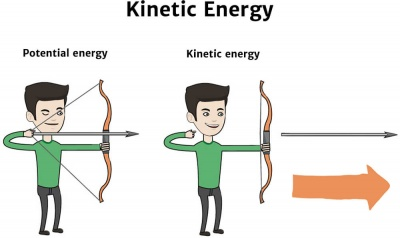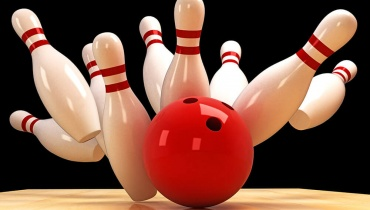# In a FlashThe potential energy of the bowstring is transferred to the arrow it's fired.

## Kinetic energy

Kinetic energy is the energy of motion. If an object is moving, it is said to have kinetic energy.

Kinetic energy is one of the two main forms of energy – the other is potential (stored) energy.

# Burning QuestionsYou can use your own movement to get other things to move.

## What is kinetic energy?

Kinetic energy is the energy of mass (an object) in motion (moving). The faster an object is moving, the more kinetic energy it has.

Any object that is moving has kinetic energy – the moving object has energy because of its motion.

## How is kinetic energy calculated?

The kinetic energy of an object is calculated based on two factors:

• Velocity: The speed the object is moving in a particular direction.

• Mass: How much matter is in the object (this is usually measured by the weight of the object).

## What is 'work'?

'Work' means a force acting on an object in the direction the object is moving in.

If we want to change the kinetic energy of a large object, i.e. move it, we need to do work on it.

Let's imagine we want to pick up a heavy object from the ground.

To lift it, we need to do 'work' to overcome the force of gravity (which is keeping it on the ground) and move the object upward.

• It will take twice as much work to lift the same object twice as high.

• If the object is twice as heavy, it will take twice as much work to lift it to the same height.

The amount of work we need to do depends on the weight of the object and the distance we want to move it.

## What are some examples of kinetic energy?

Examples of kinetic energy include:Riding a rollercoasterPeople playing sportRiding a bikeRunning water

# What Do You Mean?When a force moves something through a distance, work is done.

Kinetic energy is the energy of a moving object.

Velocity is the speed of a moving object in a particular direction.

Mass is the amount of matter container within an object.

Work is a force acting on an object in the direction of motion.

# Cool Facts

An object will always hold the same amount of kinetic energy until it speeds up or slows down.

# Cool Facts

When two pool balls collide, the kinetic energy is transferred from the moving ball to the ball it collides with.

# Cool Facts

All movable things and objects have kinetic energy whether the motion is vertical or horizontal.

# Speedy SummaryA falling house of cards has kinetic energy.

Kinetic energy is the energy of motion. If an object is moving, it has kinetic energy. The faster the motion, the more kinetic energy the object has.

# Teacher's Toolkit

Take this to the classroom!